You are on the Home/Excel/Add-Ins/Solve Polynomials/Polynomials Help pageShare Your

Installation

Solving a cubic

Solving a quartic

## Installation

The downloaded file, polynomials.zip, contains two files � polynomials.xla and polynomials.hlp. Unzip them into a directory of your choosing.

For more details see Common Installation Instructions.

You will have four new functions available in the User Defined category � Quadratic, Cubic, Quartic, and Biquadratic. You can get help for each function in the �normal� manner with the function wizard.

Returns the solution toas two numbers. Enter the formula as an array formula. Requires a minimum of two cells and four if the result is in the complex plane. For example, the two roots of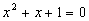are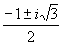, where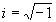.

If the formula is entered in four cells, the result will always contain complex answers.

Consider the above example. Assume the coefficients are in a spreadsheet as: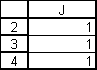The formula (entered as an array formula) is: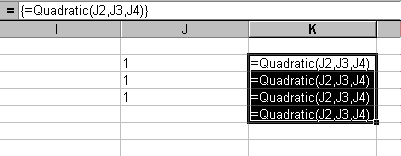Then, the result will look like: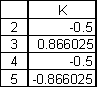## Solving a cubic

Returns the solution to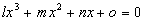as three numbers. Enter the formula as an array formula. Requires three cells if the answers are all real numbers and six if the result is in the complex plane.

If the formula is entered in six cells, the result will always contain complex answers.

## Solving a quartic

Returns the solution to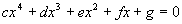as four numbers. Enter the formula as an array formula. Requires four cells if the answers are all real numbers and eight if the result is in the complex plane.

If the formula is entered in eight cells, the result will always contain complex answers.